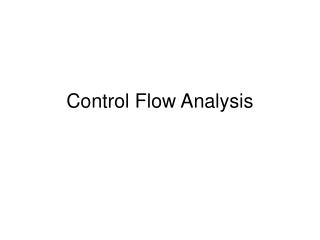# Control Flow Analysis - PowerPoint PPT PresentationDownload PresentationControl Flow Analysis

Control Flow Analysis
Download Presentation## Control Flow Analysis

- - - - - - - - - - - - - - - - - - - - - - - - - - - E N D - - - - - - - - - - - - - - - - - - - - - - - - - - -
##### Presentation Transcript

1. Control Flow Analysis

2. Control Flow Analysis • Construct representations for the structure of flow-of-control of programs • Control flow graphs represent the structure of flow-of-control within a procedure • Call graphs represent the structure of flow-of-control between procedures

3. Control Flow Graphs • Nodes in the graph are basic blocks • A basic block is a sequence of consecutive statements in which control can only enter at the beginning and leave at the end • There is an edge from a basic block b1 to a basic block b2 if control can flow from the end of b1 to the beginning of b2 ; we say b1 is a predecessor of b2 and b2 is a successor of b1

4. An Example (1) prod := 0 (2) i := 1 (3) t1 := 4 * i (4) t2 := a[t1] (5) t3 := 4 * i (6) t4 := b[t3] (7) t5 := t2 * t4 (8) t6 := prod + t5 (9) prod := t6 (10) t7 := i + 1 (11) i := t7 (12) if i <= 20 goto (3)

5. Construction of Basic Blocks • Determine the set of leaders • the first statement is a leader • the target of a jump is a leader • any statement immediately following a jump is a leader • For each leader, its basic block consists of the leader and all statements up to but not including the next leader or the end of the program

6. Representation of Basic Blocks • Each basic block is represented by a record consisting of • a count of the number of statements • a pointer to the leader • a list of predecessors • a list of successors

7. An Example (1) prod := 0 (2) i := 1 (3) t1 := 4 * i (4) t2 := a[t1] (5) t3 := 4 * i (6) t4 := b[t3] (7) t5 := t2 * t4 (8) t6 := prod + t5 (9) prod := t6 (10) t7 := i + 1 (11) i := t7 (12) if i <= 20 goto (3)

8. Call Graphs • Nodes in the graphs are procedures • There is an edge from a procedure p1 to a procedure p2 if p2 is called within p1 ; we say p1 is a predecessor of p2 and p2 is a successor of p1

9. main one two An Example procedure main( ) one( ) end procedure one( ) two( ) end procedure two( ) one( ) end